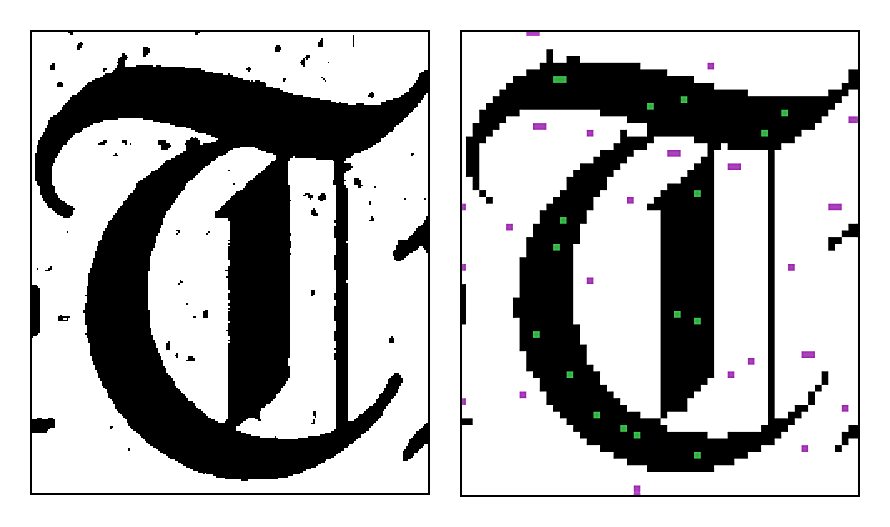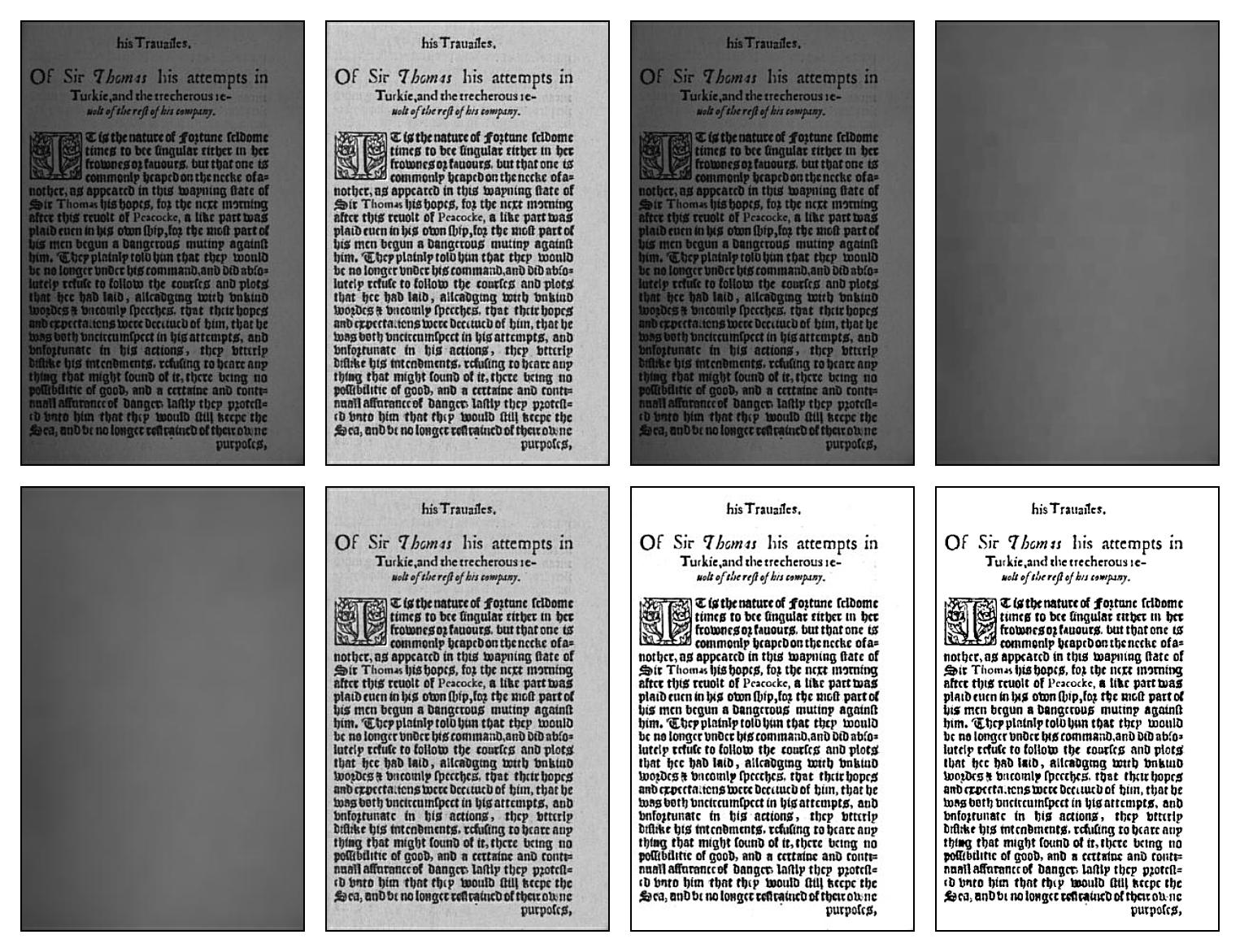# Document Image Analysis¶

date: May 20, 2010

Source material for Chapter 18 in Mathematical morphology: from theory to applications

This page describes how to run the applications and generate the figures for the Document Image Analysis chapter in Mathematical morphology: from theory to applications, edited by Laurent Najman and Hugues Talbot, ISTE-Wiley, 2010, The programs for doing this are in the open source Leptonica library.

For reference, here is a version of the chapter that includes the figures. The figures are generated by six programs:

1. livre_makefigs.c This runs the other six programs to generate all the figures.

2. livre_seedgen.c This performs the first step in an approach to page segmentation that identifies image regions by growing a seed into a mask. This generates the seed image for the image regions, which is Figure 1 in the chapter.

3. livre_pageseg.c This performs page segmentation, showing intermediate steps to identify the text and image regions. It uses a fairly complicated page image as input. It generates Figures 2–5.

4. livre_orient.c This generates Figure 6, a visual representation of the hit-miss Sels that are used for identifying the orientation of roman text, using a statistical count of ascenders and descenders.

5. livre_hmt.c This generates Figures 7 and 8, which are hit-miss Sels that are built automatically from a 1 bpp (bit/pixel) image pattern. Figures 7 and 8 were printed in grayscale. They are reproduced below in color.

6. livre_tophat.c This generates Figure 9, which shows how the tophat operation can be used to normalize and whiten the background of an image with uneven illumination.Figure 7Figure 8Extra Figure

Additionally, we give a program that generates a figure that was cut from the original paper due to length restrictions. The program, livre_adapt.c, like the tophat, compensates for nonuniform background, but in a more complicated way, by first measuring the background and then doing a locally-adaptive linear mapping in the attempt to make the background uniform. The figure demonstrates a number of operations for doing this. The eight panels are as follows:

1. The input image.

2. The background-normalized color image, where target background value is 200.

3. The input image, converted to grayscale.

4. The grayscale image closed with a 25 x 25 Sel to remove the dark text.

5. The background further smoothed by a convolution, using a 15 x 15 flat-topped block Sel.

6. The background-normalized grayscale image (again, with the target value of 200), using (3) as the input. The result in this case is very similar to (2).

7. Applying a linear TRC (tone reproduction curve) to (6), with the dark point at 30 and the white point at 180.

8. Thresholding the result to 1 bpp.

The most simple way to build these programs and generate the figures is as follows:

• Get the source code from here.

• In the src directory, type make to build the Leptonica library.

• All the programs are in the prog directory. In the prog directory, first type make.

• Then, still in the prog directory, run livre_makefigs. The figures will be placed in /tmp/, named dia_fig1.png, dia_fig2.png, etc.

The leptonica source code can also be found at code.google.com/p/leptonica. To learn about the Leptonica image processing library, read the documentation that starts here.